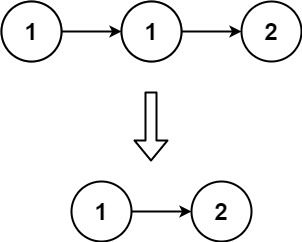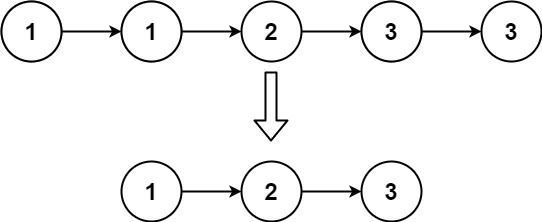# Remove Duplicates from Sorted List LeetCode Solution

Difficulty Level Easy

## Problem Statement

Remove Duplicates from Sorted List LeetCode Solution – We are given the head of a sorted linked list. We are asked to delete all the duplicates such that each element appears only once and return the linked list sorted as well.

## Examples & Explanations

Example 1:```Input: head = [1,1,2]
Output: [1,2]
Explanation: node 1 appears twice, hence it is removed```

Example 2:```Input: head = [1,1,2,3,3]
Output: [1,2,3]
Explanation: node 1 & 3 both are appearing twice and hence they are removed```

## Approach

The idea is simple, we will keep 2 pointers- a slow pointer, prev & a fast pointer, curr. If the value of curr is equal to the value of prev, it means the value is present in the linked list, hence we do not need to include curr again in the linked list, so we increment the value of curr else we increment both the pointers.

Note (Edge case): Once the curr hits NULL, we set the next of prev to NULL.

## Code

### C++ code for Remove Duplicates from Sorted List

```/**
* struct ListNode {
*     int val;
*     ListNode *next;
*     ListNode() : val(0), next(nullptr) {}
*     ListNode(int x) : val(x), next(nullptr) {}
*     ListNode(int x, ListNode *next) : val(x), next(next) {}
* };
*/
class Solution {
public:

while(curr!=NULL) {
if(prev->val == curr->val) {
curr=curr->next;
}
else {
prev->next=curr;
prev=curr;
curr=prev->next;
}
}
prev->next=NULL;
}
};```

### Java code for Remove Duplicates from Sorted List

```/**
* public class ListNode {
*     int val;
*     ListNode next;
*     ListNode() {}
*     ListNode(int val) { this.val = val; }
*     ListNode(int val, ListNode next) { this.val = val; this.next = next; }
* }
*/
class Solution {

while(curr!=null) {
if(prev.val == curr.val) {
curr=curr.next;
}
else {
prev.next=curr;
prev=curr;
curr=prev.next;
}
}
prev.next=null;